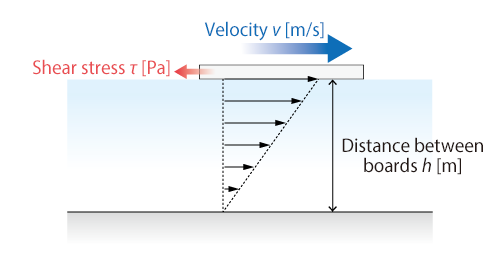#1. TOP
2. Resource Library
3. CAE Glossary
4. U-Z index
5. Viscosity coefficient

# Viscosity coefficient

## Viscosity coefficient

Viscosity coefficient is the degree of fluid viscosity.

Let us see the following example where Couette flow is considered. A fluid fills the space between two parallel wooden boards apart from each other by h [m], and the upper board is moved at the velocity of V [m/s].

Shear stress τ [Pa] acting on the board is proportional to velocity gradient V / H for most fluids. Viscosity coefficient is the proportional constant μ [Pa・s].